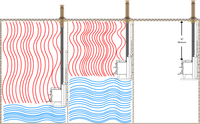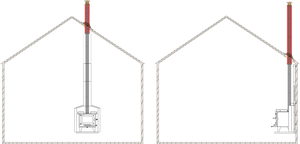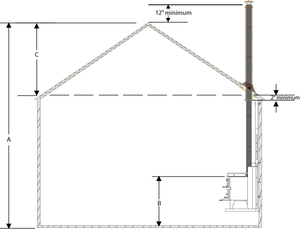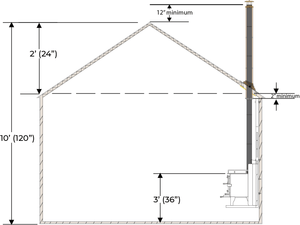## Pipe Calculation

A complete flue system requires;

• 3" double wall flue pipes ( 22.5" of usable flue pipe, 1.5" loss )
• An exit kit to exit the flue pipe ( 23" of usable flue pipe, 1" loss )
• Enough 5" insulated pipe to insulate the 3" as it goes through the ceiling, roof and to outside.
• Keep in mind that the exit kit comes with either a 24" section of 5" pipe or 2 x 12" 5" pipes. They do NOT come with any 3" pipe.

The amount of pipe needed depends on the height the stove is installed, the distance needed to pass the highest point by one foot and the placement of the stove itself.

Please see below how to calculate the pipe for a flat, round and pitched roof.## Difference Between Installation Height### Van / Flat Roof

##### 3" Double Wall Flue Pipe

A - B + 12" = Total length of 3" double wall flue pipe in inches.

Total length of 3" double wall flue pipe in inches divided by 22.5 = # of 24" long sections.

##### 5" Insulated Pipe

12" + 2" + C = Total length of 5" insulated pipe in inches.

Total length of 5" insulated pipe in inches divided by 23" = # of 24" long sections

( 12" sections available as well)### Schoolie / Curved Roof

##### 3" Double Wall Flue Pipe

A - B + 12" = Total length of 3" double wall flue pipe in inches.

Total length of 3" double wall flue pipe in inches divided by 22.5 = # of 24" long sections.

##### 5" Insulated Pipe

12" + 2" + C = Total length of 5" insulated pipe in inches.

Total length of 5" insulated pipe in inches divided by 23" = # of 24" long sections

( 12" sections available as well)### Amount of 5" Insulated Pipe Needed in relation To The Peak

The closer the pipe exits from the peak the less insulated pipe you will require.### Pitched Roof

##### 3" Double Wall Flue Pipe

A - B + 12" = Total length of 3" double wall flue pipe in inches.

Total length of 3" double wall flue pipe in inches divided by 22.5 = # of 24" long sections.

##### 5" Insulated Pipe

12" + 2" + C = Total length of 5" insulated pipe in inches.

Total length of 5" insulated pipe in inches divided by 23" = # of 24" long sections

( 12" sections available as well)

## Calculation Example### 3” double wall pipe

120” - 36” + 12”=96"

96” ÷ 22.5” = 4.26 sections

Requiring;

4 x 24” long sections

1 x 12” long section

### 5” insulated pipe.

12” + 2” + 24”= 38”

38” ÷ 23” = 1.65 sections

Requiring;

2 x 24” long sections (exit kit comes with one)